Let ψ0 and ψ2. denote respectively th...
Let ψ0 and ψ2. denote respectively the ground state and second excited state energy eigenfunction of a particle moving in a harmonic oscillator potential with frequency ψ. If at time t the particle has the wavefunction,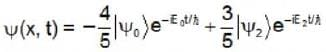The expectation value of the energy as a function of time
• a)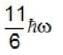• b)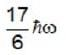• c)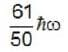• d)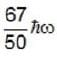Let ψ0 and ψ2. denote respectively the ground state and second...
The expectation value of an operator that does not depend on the time and commutes with the Hamiltonian is constant in time. So, (E) will remain constant with time.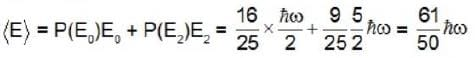1 Crore+ students have signed up on EduRev. Have you?

### Learn this topic in detailGATE Physics Mock Test Series - 6 60 Ques | 180 MinsView courses related to this question

### Quick links for GATE exam850+
Video Lectures2500+
Revision Notes600+
Online Tests10,000+
Doubts Solved
Let ψ0 and ψ2. denote respectively the ground state and second excited state energy eigenfunction of a particle moving in a harmonic oscillator potential with frequency ψ. If at time t the particle has the wavefunction,The expectation value of the energy as a function of timea)b)c)d)Correct answer is option 'C'. Can you explain this answer?
Question Description
Let ψ0 and ψ2. denote respectively the ground state and second excited state energy eigenfunction of a particle moving in a harmonic oscillator potential with frequency ψ. If at time t the particle has the wavefunction,The expectation value of the energy as a function of timea)b)c)d)Correct answer is option 'C'. Can you explain this answer? for GATE 2023 is part of GATE preparation. The Question and answers have been prepared according to the GATE exam syllabus. Information about Let ψ0 and ψ2. denote respectively the ground state and second excited state energy eigenfunction of a particle moving in a harmonic oscillator potential with frequency ψ. If at time t the particle has the wavefunction,The expectation value of the energy as a function of timea)b)c)d)Correct answer is option 'C'. Can you explain this answer? covers all topics & solutions for GATE 2023 Exam. Find important definitions, questions, meanings, examples, exercises and tests below for Let ψ0 and ψ2. denote respectively the ground state and second excited state energy eigenfunction of a particle moving in a harmonic oscillator potential with frequency ψ. If at time t the particle has the wavefunction,The expectation value of the energy as a function of timea)b)c)d)Correct answer is option 'C'. Can you explain this answer?.
Solutions for Let ψ0 and ψ2. denote respectively the ground state and second excited state energy eigenfunction of a particle moving in a harmonic oscillator potential with frequency ψ. If at time t the particle has the wavefunction,The expectation value of the energy as a function of timea)b)c)d)Correct answer is option 'C'. Can you explain this answer? in English & in Hindi are available as part of our courses for GATE. Download more important topics, notes, lectures and mock test series for GATE Exam by signing up for free.
Here you can find the meaning of Let ψ0 and ψ2. denote respectively the ground state and second excited state energy eigenfunction of a particle moving in a harmonic oscillator potential with frequency ψ. If at time t the particle has the wavefunction,The expectation value of the energy as a function of timea)b)c)d)Correct answer is option 'C'. Can you explain this answer? defined & explained in the simplest way possible. Besides giving the explanation of Let ψ0 and ψ2. denote respectively the ground state and second excited state energy eigenfunction of a particle moving in a harmonic oscillator potential with frequency ψ. If at time t the particle has the wavefunction,The expectation value of the energy as a function of timea)b)c)d)Correct answer is option 'C'. Can you explain this answer?, a detailed solution for Let ψ0 and ψ2. denote respectively the ground state and second excited state energy eigenfunction of a particle moving in a harmonic oscillator potential with frequency ψ. If at time t the particle has the wavefunction,The expectation value of the energy as a function of timea)b)c)d)Correct answer is option 'C'. Can you explain this answer? has been provided alongside types of Let ψ0 and ψ2. denote respectively the ground state and second excited state energy eigenfunction of a particle moving in a harmonic oscillator potential with frequency ψ. If at time t the particle has the wavefunction,The expectation value of the energy as a function of timea)b)c)d)Correct answer is option 'C'. Can you explain this answer? theory, EduRev gives you an ample number of questions to practice Let ψ0 and ψ2. denote respectively the ground state and second excited state energy eigenfunction of a particle moving in a harmonic oscillator potential with frequency ψ. If at time t the particle has the wavefunction,The expectation value of the energy as a function of timea)b)c)d)Correct answer is option 'C'. Can you explain this answer? tests, examples and also practice GATE tests.(Scan QR code)